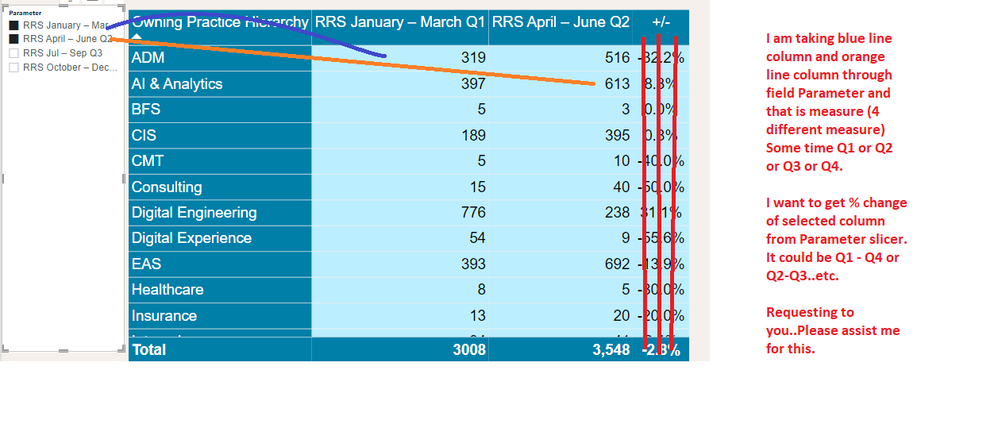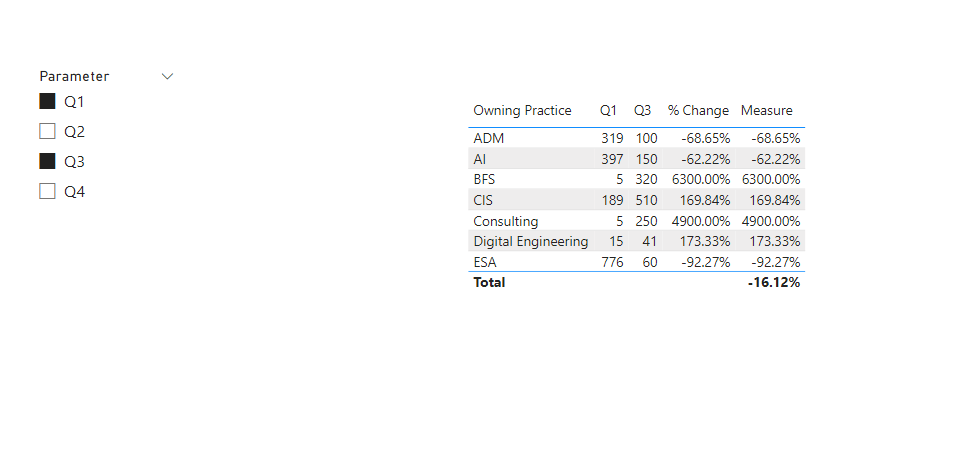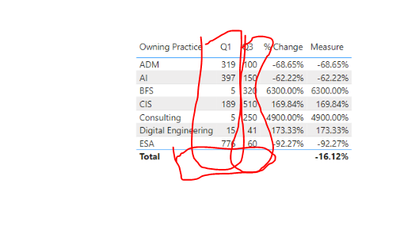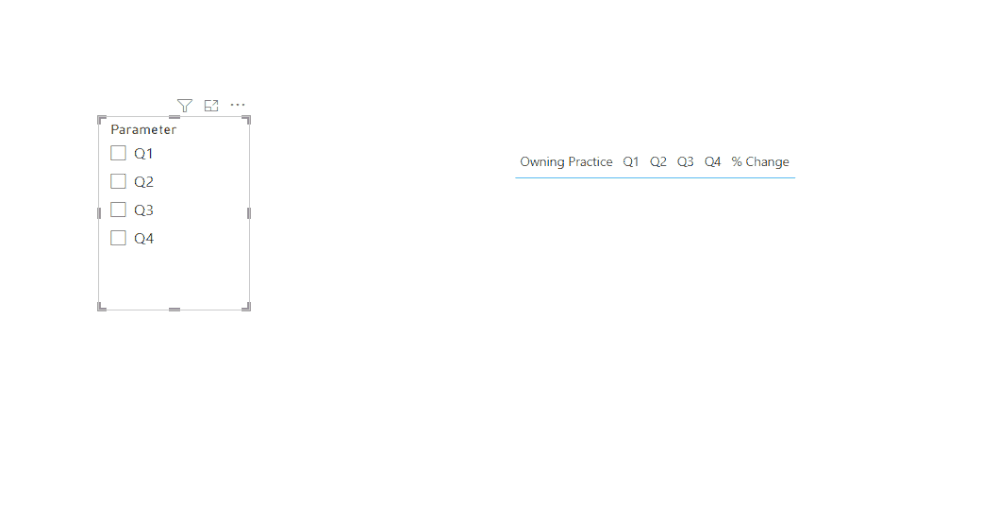cancel
Showing results for
Did you mean:Frequent Visitor

## Need Help on Dax - % Change

Dear Team

Good Morning / Good Evening / Good Afternoon.

I have asked question like this before however this time with new requirement.

I need % change for dynamic column change value.

(As per given image)

I am taking blue line column and orange line column through field Parameter and that is measure (4 different measure)
Some time Q1 or Q2 or Q3 or Q4.

I want to get % change of selected column from Parameter slicer.
It could be Q1 - Q4 or Q2-Q3 or Q3-Q4 or Q1 - Q3..etc.

Requesting to you..Please assist me for this.Please assist.

Thanks

Satya Prakash Pandey

1 ACCEPTED SOLUTIONCommunity Support

Just need adjust the dax formula simply, please try below dax formual:

``````Measure =
VAR _str =
CONCATENATEX ( SELECTCOLUMNS ( Parameter, "Test", [Parameter] ), [Test] )
VAR cur_own =
SELECTEDVALUE ( 'Table 1'[Owning Practice] )
VAR _a =
CALCULATE ( MAX ( 'Table 1'[Q1] ), 'Table 1'[Owning Practice] = cur_own )
VAR _b =
CALCULATE ( MAX ( 'Table 1'[Q2] ), 'Table 1'[Owning Practice] = cur_own )
VAR _c =
CALCULATE ( MAX ( 'Table 1'[Q3] ), 'Table 1'[Owning Practice] = cur_own )
VAR _d =
CALCULATE ( MAX ( 'Table 1'[Q4] ), 'Table 1'[Owning Practice] = cur_own )
VAR _e =
SUMX ( ALL ( 'Table 1' ), [Q1] )
VAR _f =
SUMX ( ALL ( 'Table 1' ), [Q2] )
VAR _g =
SUMX ( ALL ( 'Table 1' ), [Q3] )
VAR _h =
SUMX ( ALL ( 'Table 1' ), [Q4] )
VAR s1 = "Q1Q2"
VAR s2 = "Q1Q3"
VAR s3 = "Q1Q4"
VAR s4 = "Q2Q3"
VAR s5 = "Q2Q4"
VAR s6 = "Q3Q4"
VAR _val =
SWITCH (
_str,
s1,
( _b - _a ) / _a,
s2,
( _c - _a ) / _a,
s3,
( _d - _a ) / _a,
s4,
( _c - _b ) / _b,
s5,
( _d - _c ) / _b,
s6,
( _d - _c ) / _c
)
VAR _val2 =
SWITCH (
_str,
s1,
( _f - _e ) / _e,
s2,
( _g - _e ) / _e,
s3,
( _h - _e ) / _e,
s4,
( _g - _f ) / _f,
s5,
( _h - _f ) / _f,
s6,
( _h - _g ) / _g
)
RETURN
IF ( HASONEVALUE ( 'Table 1'[Owning Practice] ), _val, _val2 )
``````Please refer the attached .pbix file.

Best regards,
Community Support Team_Binbin Yu
If this post helps, then please consider Accept it as the solution to help the other members find it more quickly.

5 REPLIES 5Frequent Visitor

Thanks for the help. It really worked for me.

However can you help for total of the column and total % change of the % change column as its not coming .Community Support

Just need adjust the dax formula simply, please try below dax formual:

``````Measure =
VAR _str =
CONCATENATEX ( SELECTCOLUMNS ( Parameter, "Test", [Parameter] ), [Test] )
VAR cur_own =
SELECTEDVALUE ( 'Table 1'[Owning Practice] )
VAR _a =
CALCULATE ( MAX ( 'Table 1'[Q1] ), 'Table 1'[Owning Practice] = cur_own )
VAR _b =
CALCULATE ( MAX ( 'Table 1'[Q2] ), 'Table 1'[Owning Practice] = cur_own )
VAR _c =
CALCULATE ( MAX ( 'Table 1'[Q3] ), 'Table 1'[Owning Practice] = cur_own )
VAR _d =
CALCULATE ( MAX ( 'Table 1'[Q4] ), 'Table 1'[Owning Practice] = cur_own )
VAR _e =
SUMX ( ALL ( 'Table 1' ), [Q1] )
VAR _f =
SUMX ( ALL ( 'Table 1' ), [Q2] )
VAR _g =
SUMX ( ALL ( 'Table 1' ), [Q3] )
VAR _h =
SUMX ( ALL ( 'Table 1' ), [Q4] )
VAR s1 = "Q1Q2"
VAR s2 = "Q1Q3"
VAR s3 = "Q1Q4"
VAR s4 = "Q2Q3"
VAR s5 = "Q2Q4"
VAR s6 = "Q3Q4"
VAR _val =
SWITCH (
_str,
s1,
( _b - _a ) / _a,
s2,
( _c - _a ) / _a,
s3,
( _d - _a ) / _a,
s4,
( _c - _b ) / _b,
s5,
( _d - _c ) / _b,
s6,
( _d - _c ) / _c
)
VAR _val2 =
SWITCH (
_str,
s1,
( _f - _e ) / _e,
s2,
( _g - _e ) / _e,
s3,
( _h - _e ) / _e,
s4,
( _g - _f ) / _f,
s5,
( _h - _f ) / _f,
s6,
( _h - _g ) / _g
)
RETURN
IF ( HASONEVALUE ( 'Table 1'[Owning Practice] ), _val, _val2 )
``````Please refer the attached .pbix file.

Best regards,
Community Support Team_Binbin Yu
If this post helps, then please consider Accept it as the solution to help the other members find it more quickly.Frequent Visitor

Hi Sir
Thnks for the support..However may you help to get total of below
Q1 or Q2 or Q3 or Q4Frequent Visitor

Hi Sir..

All is worked however I want to total of first two columns..which is not coming currently.Community Support

1. because you don't provide sample data, i create a test table for test according to your descriptions

Table:2. create a measure with below dax formula

``````% Change =
VAR _str =
CONCATENATEX ( SELECTCOLUMNS ( Parameter, "Test", [Parameter] ), [Test] )
VAR cur_own =
SELECTEDVALUE ( 'Table 1'[Owning Practice] )
VAR _a =
CALCULATE ( MAX ( 'Table 1'[Q1] ), 'Table 1'[Owning Practice] = cur_own )
VAR _b =
CALCULATE ( MAX ( 'Table 1'[Q2] ), 'Table 1'[Owning Practice] = cur_own )
VAR _c =
CALCULATE ( MAX ( 'Table 1'[Q3] ), 'Table 1'[Owning Practice] = cur_own )
VAR _d =
CALCULATE ( MAX ( 'Table 1'[Q4] ), 'Table 1'[Owning Practice] = cur_own )
VAR s1 = "Q1Q2"
VAR s2 = "Q1Q3"
VAR s3 = "Q1Q4"
VAR s4 = "Q2Q3"
VAR s5 = "Q2Q4"
VAR s6 = "Q3Q4"
RETURN
SWITCH (
_str,
s1,
( _b - _a ) / _a,
s2,
( _c - _a ) / _a,
s3,
( _d - _a ) / _a,
s4,
( _c - _b ) / _b,
s5,
( _d - _c ) / _b,
s6,
( _d - _c ) / _c
)
``````

3. add a table visual with fields and measureBest regards,
Community Support Team_Binbin Yu
If this post helps, then please consider Accept it as the solution to help the other members find it more quickly.